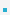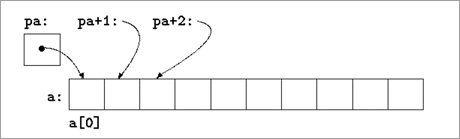4. Pointer ArithmeticSuppose int *pa = a;Pointer not an int, but can add or subtract an int from a pointer: pa + i points to a[i]

 *(pa+i)      // the contents of a[i]Address value increments by i times size of data type Suppose a has address 100. Then a has    address 112.Suppose char *pc= (char *)pa.
– What value of i satisfies (int *)(pc+i) == pa + 3?